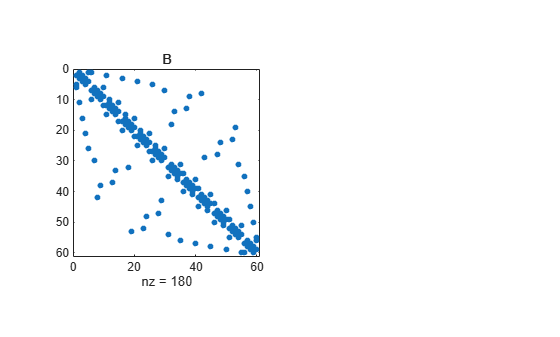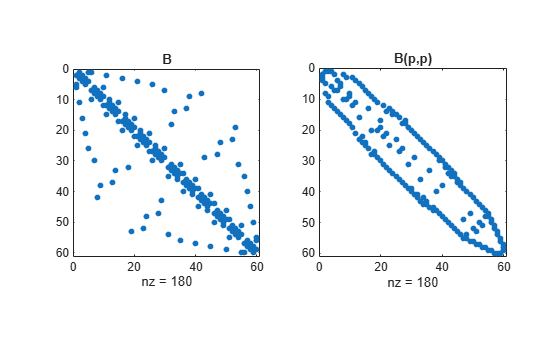# symrcm

Sparse reverse Cuthill-McKee ordering

## Syntax

```r = symrcm(S) ```

## Description

`r = symrcm(S)` returns the symmetric reverse Cuthill-McKee ordering of `S`. This is a permutation `r` such that `S(r,r)` tends to have its nonzero elements closer to the diagonal. This is a good preordering for LU or Cholesky factorization of matrices that come from long, skinny problems. The ordering works for both symmetric and nonsymmetric `S`.

For a real, symmetric sparse matrix, `S`, the eigenvalues of `S(r,r)` are the same as those of `S`, but `eig(S(r,r))` probably takes less time to compute than `eig(S)`.

## Examples

collapse all

The statement

`B = bucky;`

uses a function in the `demos` toolbox to generate the adjacency graph of a truncated icosahedron. This is better known as a soccer ball, a Buckminster Fuller geodesic dome (hence the name `bucky`), or, more recently, as a 60-atom carbon molecule. There are 60 vertices. The vertices have been ordered by numbering half of them from one hemisphere, pentagon by pentagon; then reflecting into the other hemisphere and gluing the two halves together.

With this numbering, the matrix does not have a particularly narrow bandwidth, as the first spy plot shows:

```figure(); subplot(1,2,1),spy(B),title('B')```The reverse Cuthill-McKee ordering is obtained with:

```p = symrcm(B); R = B(p,p);```

The `spy` plot shows a much narrower bandwidth.

`subplot(1,2,2),spy(R),title('B(p,p)')`This example is continued in the reference page for `symamd`.

The bandwidth can also be computed with:

```[i,j] = find(B); bw = max(i-j) + 1;```

The bandwidths of `B` and `R` are 35 and 12, respectively.

## Algorithms

The algorithm first finds a pseudoperipheral vertex of the graph of the matrix. It then generates a level structure by breadth-first search and orders the vertices by decreasing distance from the pseudoperipheral vertex. The implementation is based closely on the SPARSPAK implementation described by George and Liu.

## References

 George, Alan and Joseph Liu, Computer Solution of Large Sparse Positive Definite Systems, Prentice-Hall, 1981.

 Gilbert, John R., Cleve Moler, and Robert Schreiber, “Sparse Matrices in MATLAB: Design and Implementation,” SIAM Journal on Matrix Analysis, 1992. A slightly expanded version is also available as a technical report from the Xerox Palo Alto Research Center.